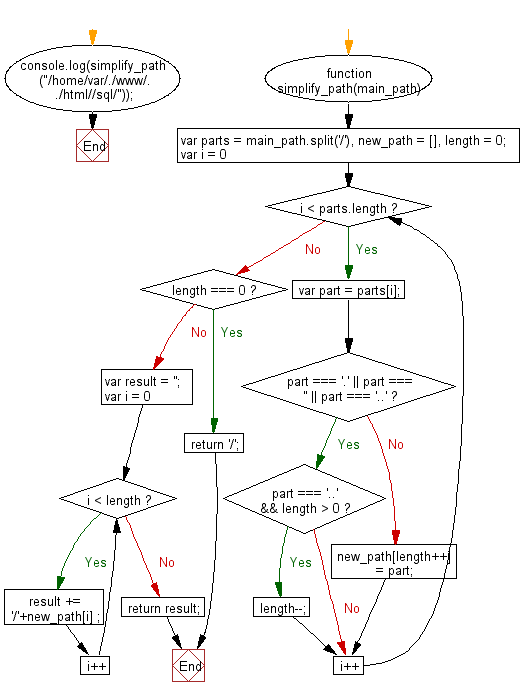# JavaScript: Simplify a given absolute path for a file in Unix-style

## JavaScript Basic: Exercise-142 with Solution

Write a JavaScript program to simplify a given absolute path for a file in Unix-style.

Sample Solution:

HTML Code:

``````<!DOCTYPE html>
<html>
<meta charset="utf-8">
<meta name="viewport" content="width=device-width">
<title>Simplify a given absolute path for a file in Unix-style.</title>
<body>

</body>
</html>
```
```

JavaScript Code:

``````function simplify_path(main_path) {
var parts = main_path.split('/'),
new_path = [],
length = 0;
for (var i = 0; i < parts.length; i++) {
var part = parts[i];
if (part === '.' || part === '' || part === '..') {
if (part === '..' && length > 0) {
length--;
}
continue;
}
new_path[length++] = part;
}

if (length === 0) {
return '/';
}

var result = '';
for (var i = 0; i < length; i++) {
result +=  '/'+new_path[i] ;
}

return result;
}
console.log(simplify_path("/home/var/./www/../html//sql/"));
``````

Sample Output:

```/home/var/html/sql
```

Flowchart:ES6 Version:

``````function simplify_path(main_path) {
const parts = main_path.split('/');
const new_path = [];
let length = 0;
for (var i = 0; i < parts.length; i++) {
const part = parts[i];
if (part === '.' || part === '' || part === '..') {
if (part === '..' && length > 0) {
length--;
}
continue;
}
new_path[length++] = part;
}

if (length === 0) {
return '/';
}

let result = '';
for (var i = 0; i < length; i++) {
result +=  `/\${new_path[i]}` ;
}

return result;
}
console.log(simplify_path("/home/var/./www/../html//sql/"));
``````

Live Demo:

See the Pen javascript-basic-exercise-142 by w3resource (@w3resource) on CodePen.

Improve this sample solution and post your code through Disqus

What is the difficulty level of this exercise?

Test your Programming skills with w3resource's quiz.

﻿

## JavaScript: Tips of the Day

Returns the sum of the powers of all the numbers from start to end (both inclusive)

Example:

```const sumPower = (end, power = 2, start = 1) =>
Array(end + 1 - start)
.fill(0)
.map((x, i) => (i + start) ** power)
.reduce((a, b) => a + b, 0);
console.log(sumPower(10)); // 385
console.log(sumPower(10, 3)); // 3025
console.log(sumPower(10, 3, 5)); // 2925
```

Output:

```385
3025
2925
```